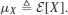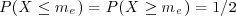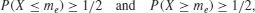5.14 LOCATION PARAMETERS: MEAN, MEDIAN, AND MODE

A parameter that characterizes the center and location of a pdf is useful as a simple single descriptor of a random variable, as was used in Chapter 3 when we described the properties of several distributions. There are three such measures that generally give different values, although they coincide for some distributions.

Definition: Mean The mean of random variable X is its expectation:

(5.185)The mean of a random variable can be viewed as that value obtained by averaging the outcomes over a very large number of experiments. It can also be viewed functionally as the area of FX(x) weighted by the odd function g(x) = x which places increasing emphasis on larger values (positive and negative) of x.

Definition: Median The median me of continuous random variable X is the midpoint of the cdf:

(5.186)which is equivalent to. For a discrete random variable, this last expression is modified to two conditions:

(5.187)where the directions of the inequalities relative to 1/2 are the same. Substituting the pdfs yields

(5.188)

Get Probability, Random Variables, and Random Processes: Theory and Signal Processing Applications now with the O’Reilly learning platform.

O’Reilly members experience books, live events, courses curated by job role, and more from O’Reilly and nearly 200 top publishers.